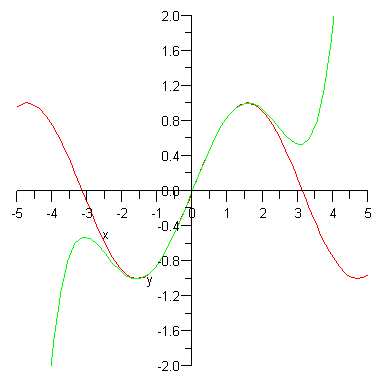# Prime equals triple prime?

Calculus Level 2$f'(x) = f'''(x)$

For some infinitely differentiable function $f(x)$, it satisfies the above equation. Given the initial conditions

$f(1) = 6, f'(1) = 5, f''(1) = -3$

What is the value of $f( \ln(42) )$?

Details and Assumptions

$f'(k), f''(k), f'''(k)$ denote the first, second, and third derivative of $f(x)$ at $x=k$ respectively.

×